# GSEB Solutions Class 12 Chemistry Chapter 2 Solutions

Gujarat Board GSEB Textbook Solutions Class 12 Chemistry Chapter 2 Solutions Textbook Questions and Answers, Additional Important Questions, Notes Pdf.

## Gujarat Board Textbook Solutions Class 12 Chemistry Chapter 2 Solutions

### GSEB Class 12 Chemistry Solutions InText Questions and Answers

Question 1.
Calculate the mass percentage of benzene (C6H6) and carbon tetrachloride (CCl4) if 22 g of benzene is dissolved in 122 g of carbon tetrachloride.
Mass percentage of benzene
=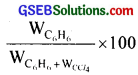= $$\frac{22}{22+122}$$ × 100 = 15.28%
Mass percentage of CCl4 = 100 – mass percentage of benzene = 100 – 15.28 = 84.72%Question 2.
Calculate the mole fraction of benzene in the solution containing 30% by mass in carbon tetrachloride.
Let the mass of solution be 100 g
∴ Mass of C6H6 = 30g
Mass of CCl4 = 100 – 30 = 70 g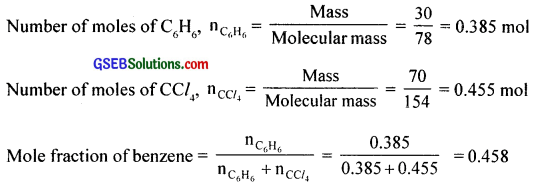Mole fraction of CCl4 = 1 – 0.458 = 0.542

Question 3.
Calculate the molarity of each of the following solutions:
(a) 30 g of Co(NO3)2. 6H2O in 4.3 L of solution.
(b) 30 ml of 0.5 M H2SO4 diluted to 500 ml.
(a) Formula mass of Co(NO3)2.6H2O, MB = 291
Molarity = $$\frac{W_{B}}{M_{B} \times V \text { in } L}$$ = $$\frac{30}{291×4.3}$$
= 0.023 M
(b) M1 = 0.5 M, V1 = 30 ml
M2 = ?, V2 = 500 ml
M1V1 = M2V2
∴ M2 = $$\frac { M_{ 1 }V_{ 1 } }{ V_{ 2 } }$$
= $$\frac{0.5×30}{500}$$
= 0.03 MQuestion 4.
Calculate the mass of urea (NH2CONH2) required in making 2.5 kg of 0.25 molal aqueous solution?
0.25 molal aqueous solution means that
Moles of urea = 0.25 mole
Mass of solvent (water) = 1 kg = 1000 g
Molar mass of urea = 14 + 2 + 12 + 16 + 14 + 2 = 60g mol-1
∴ 0.25 mole of urea = 60 × 0.25 mole = 15 g
Total mass of the solution = 1000 + 15 g = 1015 g = 1.015 g
∵ 1.015 kg of solution contain urea = 15 g
∴ 2.5 kg of solution will require urea = $$\frac { 15 }{ 1.015 }$$ × 2.5 kg = 37g .

Question 5.
Calculate (a) molality, (b) molarity and (c) mole fraction of KI if the density of 20% (mass/mass) aqueous KI is 1.202 g mL-1.
Let the mass of the solution be 100 g.
∴ Mass of KI, WB = 20 g
Mass of water, WA = 100 – 20 = 80 g
MB = 166 gQuestion 6.
H2S, a toxic gas with rotten egg like smell, is used for the qualitative analysis. If the solubility of H2S in water at STP is 0.195 m, calculate Henry’s law constant?
Molality, 0.195 m means 0.195 mole of H2S in 100 g of water.
1000 g water = $$\frac{1000}{18}$$ = 55.5 mol
∴ Mole fraction of H2S = $$\frac{0.195}{0.195+55.5}$$ = 0.0035
Henry’s constant, KH = $$\frac{\text { Pressure }}{\text { Mole fraction }}$$
Pressure = 1 bar
∴ KH = $$\frac{1 bar}{0.0035}$$ = 285.9 barQuestion 7.
Henry’s law constant for CO2 in water is 1.67 × 108 Pa at 298 K. Calculate the quantity of CO2 in 500 ml of soda water when packed under 2.5 atm CO2 pressure at 298 K?
According to Henry’s law, p = KHX
Mole fraction, X = $$\frac { p }{ K_{ H } }$$ = $$\frac{2.5 \text { atm }}{1.67 \times 10^{8} \mathrm{~Pa}}$$
= $$\frac{2.5 \times 10^{5} \mathrm{~Pa}}{1.67 \times 10^{8} \mathrm{~Pa}}$$
= 0.0015
In the given solution, amount of water = 500 ml = 500 g
Number of moles of water, $$\mathrm{n}_{\mathrm{H}_{2} \mathrm{O}}$$ = $$\frac{500}{18}$$ = 27.78 mol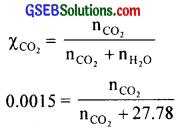$$\mathrm{n}_{\mathrm{CO}_{2}}$$ = 0.417 × 44 = 1.836 g

Question 8.
The vapour pressure of pure liquids A and B are 450 and 700 mm Hg respectively, at 350 K. Find out the composition of the liquid mixture if total vapour pressure is 600 mm Hg. Also find the composition of the vapour phase?
P0A = 450 mm Hg, P0B = 700 m Hg, Ptotal = 600 mm Hg
Ptotal = xAP0A + xBP0B
600 = xA (450) + xB (700) = xA (450) + (1 – xA) 700
xA = 0.4
xB = 1 – 0.4 = 0.6
600 = xA(450) + xB(700) = xA(450) + (1 – xA)700
xA = 0.4
xB = 1 – 0.4 = 0.6
In vapour phase, PA = 0.4 × 450 = 180 mm Hg
PB = 0.6 × 700 = 420 mm Hg
∴ x’A = $$\frac{180}{180+420}$$ = 0.3
x’B = $$\frac{420}{180+420}$$ = 0.7Question 9.
Vapour pressure of pure water at 298 K is 23.8 mm Hg. 50 g of urea (NH2CONH2) is dissolved in 850 g of water. Calculate the vapour pressure of water for this solution and its relative lowering.
$$\mathrm{n}_{\mathrm{H}_{2} \mathrm{O}}$$ = $$\frac{850}{18}$$ = 47.22
nH2O = $$\frac{50}{60}$$ = 0.83
Xwater = $$\frac{47.22}{47.22+0.83}$$ = 0.9827
P = P0 × Xwater = 23.8 × 0.9827 = 23.39 mm Hg
Relative lowering of vapour pressure = Xurea = 1 – Xwater
= 1 – 0.9827 = 0.0173

Question 10.
Boiling point of water at 750 mm Hg is 99.63°C. How much sucrose is to be added to 500 g of water such that it boils at 100°C?
Molecular mass of sucrose, MB = 342
kb = 0.52
∆Tb = 100 – 99.63 = 0.37 k
WA = 500 gQuestion 11.
Calculate the mass of ascorbic acid (Vitamin C, C6H8O6) to be dissolved in 75 g of acetic acid to lower its melting point by 1.5°C. Kf = 3.9 K kg mol-1.
Molecular mass of ascorbic acid, MB = 176
WA = 75 g, ∆Tf = 1.5, Kf = 3.9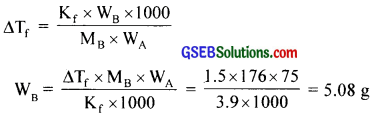Question 12.
Calculate the osmotic pressure in pascals exerted by a solution prepared by dissolving 1.0 g of polymer of molar mass 185,000 in 450 ml of water at 37°C.
WB = 1g, MB = 1850000, V = 450 ml = 0.45L, T = 37°C = 310 K
R = 0.083 L bar K-1 mol-1### GSEB Class 12 Chemistry Solutions Text Book Questions and Answers

Question 1.
Define the term solution. How many types of solutions are formed? Write briefly about each type with an example?
A true solution is a homogeneous mixture of two or more substances. The constituent which is in larger amount is called a solvent and that in smaller quantity is called a solute.

Since the solvent and solute may be either gaseous, liquid or solid, the number of possible types of binary solutions that can be prepared are given below.Question 2.
Suppose a solid solution is formed between two substances. One of whose particles are very large and the other particles are very small. What kind of solid solution is this likely to be?
Solid in solid type. eg. Copper in gold. This type of solutions are called alloys.Question 3.
Define the following terms:

1. Mole fraction
2. Molality
3. Molarity
4. Mass percentage

1. Mole fraction (X):
The mole fraction of any component in a solution is the ratio of the number of moles of that component to the sum of the number of moles of all the components present in thei solution.
For a binary solution containing A and B,where nA and nB are the numbers of moles of components A and B respectively.

2. Molality (m):
Molality is the number of moles of the solute present per kilogram (1000 g) of the solvent. The unit of molality ¡s moles per kg or m (molal).3. Molarity (M):
Molarity is the number of moles of the solute present per litre of the solution.
The unit of molarity is moles per litre or M (molar).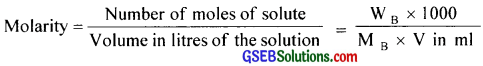4. Mass percentage ($$\frac{w}{W}$$): The mass of a component present in 100 mass unit of the solution is called its mass percentage. It has no unit.Question 4.
Concentrated nitric acid used in laboratory works is 68% nitric acid by mass in aqueous solution. What should be the molarity of such a sample of the acid if the density of the solution is 1.504 g ml-1?
Mass of 1 litre of solution = density × volume
= 1.504 × 1000 = 1504 g
Mass of 68% HNO3 = $$\frac{68×1504}{100}$$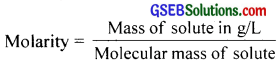= $$\frac{68×1504}{100×63}$$
= 16.23 M

Question 5.
A solution of glucose in water is labelled as 10% w/W, what would be the molality and mole fraction of each component in the solution? If the density of solution is 1.2 g ml-1, then what shall be the molarity of the solution?
For a 10% ($$\frac{w}{W}$$) solution of glucose, mass of glucose = $$\frac{10}{100}$$ × 1000 = 100 g/kg and mass of water = 900 g/kgMole fraction of water = 1 – mole fraction of glucose = 1 – 0.01 = 0.99

Question 6.
A solution is obtained by mixing 300 g of 25% solution and 400 g of 40% solution by mass. Calculate the mass percentage of the resulting solution?
Mass of solute in 300 g of 25% solution = $$\frac{25}{100}$$ × 300 = 75 g
Mass of solute in 400 g of 40% solution = $$\frac{40}{100}$$ × 400 = 160 g
Total mass of solute = 75 + 160 = 235 g
Total mass of solution = 700 g
Mass percentage of solute = $$\frac{235}{700}$$ × 100 = 33.5%
Mass percentage of solvent = 100 – 33.5 = 66.5%Question 7.
An antifreeze solution is prepared from 222.6 g of ethylene glycol (C2H6O2) and 200 g of water. Calculate the molality of the solution. If the density of the solution is 1.072 g ml-1 then what shall be the molarity of the solution?Question 8.
A sample of drinking water was found to be severely contaminated with chloroform (CHCl3) supposed to be a carcinogen. The level of contamination was 15 ppm (by mass):

1. Express this in percent by mass
2. Determine the molality of chloroform in the water sample.

1. 15 ppm = $$\frac{15}{10^{6}}$$ × 100 = 15 × 10-4 % by mass

2. Molality = $$\frac{W_{B} \times 1000}{M_{B} \times W_{A}}$$
= $$\frac{15 \times 10^{-6} \times 1000}{118.5 \times 1}$$
= 1.26 × 10-4 m

Question 9.
What role does the molecular interaction play in a solution of alcohol and water?
Water and alcohol molecules interact very strongly with each other through hydrogen bonding and make them completely miscible.

Question 10.
Why do gases always tend to be less soluble in liquids as the temperature is raised?
As the temperature increases, the kinetic energy of gaseous molecules increases and their bonds with liquid solvent break and escape into the vapour phase. Gases are less soluble in liquids as temperature is raised.

Question 11.
State Henry’s law and mention some important applications?
Henry’s law:
It states that at constant temperature, the solubility of a gas in a liquid is directly proportional to the pressure of the gas.

Applications:

1. It is used
• In the production of carbonated beverages.
• In the deep sea diving.
• In the function of lungs.Question 12.
The partial pressure of ethane over a solution containing 6.56 × 10-3 g of ethane is 1 bar. If the solution contains 5.00 × 10-2 g of ethane, then what shall be the partial pressure of the gas?
From Henry’s law, m = KHp
In the first case, KH = $$\frac{m}{p}$$ = $$\frac { 6.56\times 10^{ -3 } }{ 1 }$$
= 6.56 × 10-3 g bar-1
In the second case, p = $$\frac{\mathrm{m}}{\mathrm{K}_{\mathrm{H}}}$$
= $$\frac { 5.00\times 10^{ -2 }g }{ (6.56\times 10^{ -3 }g bar^{ -1 })\quad }$$ = 7.62 bar

Question 13.
What is meant by positive and negative deviations from Raoult’s law and how is the sign of ∆minH related to positive and negative deviations from Raoult’s law?
A non-ideal solution is one which does not obey Raoult’s law. For ideal solutions,
(I) PA ≠ XAP0A and PB ≠ XBP0B
(II) ∆mixing V ≠ 0
(III) ∆mixing H ≠ 0

Based on the nature of deviation from Raoults’ law, non-ideal solutions are divided into two types.
1. non-ideal solutions with positive deviation and
2. non-ideal solutions with negative deviation.

1. Non-ideal solutions which show positive deviation:
In this case the partial vapour pressure of any component of the solution and the total vapour pressure of solution are greater than that expected from Raoult’s law. i.e., PA > XAP0A, PB > XBP0B and PS > XAP0A + XBP0B. In these solutions, ∆mixingH and ∆mixing V are positive.

Cause of positive deviation:
Positive deviation is shown by liquid pairs for which A – B force of attraction is eaker than A – A and B – B forces of attractions. As a result, the escaping tendency of the molecules will be greater than that in the pure solutions. Thus, the partial pressure of both of them over the solution are greater than that predicted by Raoult’s law and hence the solution exhibits a large vapour pressure than that expected.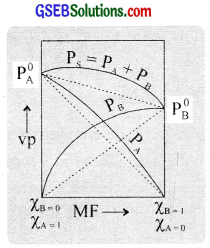Due to the decrease in attractive forces, energy is increased. i.e., the process is endothermic. Thus ∆mixing H > 0 (+ ve). Because of the
decrease in attraction, the particles are loosely held and hence there is an increase in volume. Thus ∆mixing V > 0 (+ve). e.g. A solution of ethanol and cyclohexane. In pure ethanol molecules, there arc intermolecular hydrogen bonds.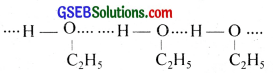When cyclohexane is added to ethanol, the cyclohexane molecules get placed in between the ethanol molecules, thereby breaking the hydrogen bonds. This reduces the intermolecular attraction. As a result, ∆mixing V and ∆mixing H are positive. Solutions such as,

• acetone and CS2
• acetone and alcohol
• acetone and benzene
• water and alcohol
• ether and acetone etc. show positive deviation

2. Non – ideal solutions show negative deviations:
In this case the partial vapour pressure of any component of the solution and the total vapour pressure of the solution are less than that expected from Raoult’s law. i.e; PA < XAP0A, PB < XBP0B and Ps < XAP0B. In these solutions ∆mixingH and ∆mixing V are negative.

Cause of negative Jeviation:
Negative deviation is shosn by liquid pairs of which A – B threes of attraction is stronger than A – A and B – B forces olatiractions. Thus, in the solution the solute and solvent parteles are held more tightly thaii that in the pure components. Due to this, the escaping tendency of the molecules of both the components will be less in the solution than in the pure components. Conscquently, the partial pressures of both the components are less titan that expected from Raoult’s law and hence the solution exhibits a less vapour pressure than that expected.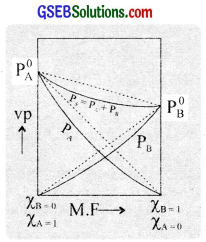Due to increase in attractive forces. energy is decreased, i.e., the process is exoththermic. Thus ∆mixing H < 0 (- ve). Because of the increase in attraction, the particles are tightly held and hence there is a decrease in oIume. Thus ∆mixing V < O (- ve). e.g. A solution of chloroform and acetone. When these two are mixed. hydrogen bonding occurs heteen the to molecular species. This increases the intermolecular attraction as illustrated below.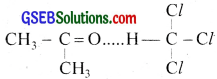This attraction brings their molecules closer thereby reducing the volume. Solutions such as,

• chloroform and ethers
• beniene and chloroform
• water and nitric acid
• acetone and aniline
• chlorotorm and nitric acid etc. also show negative deviation.

Azeotropic mixtures:
Mixtures of two liquids which boil ai a constant temperature and can he distilled without any change in their composition arc culled azeotropic mixtures. These are formed by non-ideal solutions and are of two types.

1. Maximum boiling azeotropes:
These are mixtures of two liquids whose boiling points are more than either of the tsso pure components. These are formed by non-ideal solutions showing negative devialitms e.g. HNO3 (68 %) + water (32 %) mixture boils at 393.5 K.

2. Minimum boiling azeotropes:
These are mixtures of two liquids whose boiling points are less than either of the two components. These are formed by non-ideal solutions showing positive deviations. e.g. Ethanol (95.6 %) + water (4.4 %) mixture boils at 351.15 K.Question 14.
An aqueous solution of 2% non-volatile solute exerts a pressure of 1.004 bar at the normal boiling point of the solvent. What is the molar mass of the solute?
WA = 100 – 2 = 98 g,
WB = 2,
MA = 18,
P0A = 1.013 bar,
PA = 1.004 bar.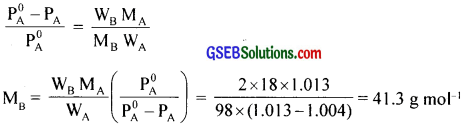Question 15.
Heptane and octane form an ideal solution. At 373 K, the vapour pressures of the two liquid components are 105.2 kPa and 46.8 kPa respectively. What will be the vapour pressure of a mixture of 26.0 g of heptane and 35 g of octane?
P0heptane = 105.2 kPa, P0octane = 46.8 kPa
Moles of heptane = $$\frac{26}{100}$$ = 0.26, Moles of octane = $$\frac{35}{114}$$ = 0.307
Mole fraction of heptane = $$\frac{0.26}{0.26+0.307}$$ = 0.458
Mole fraction of octane = 1 – 0.458
= 0.542
Vapour pressure due to heptane = mole fraction × P0heptane = 0.458 × 105.2
= 48.18 kPa
Vapour pressure due to octane = 0.542 × 46.8 = 25.36 kPa
Total vapour pressure = 48.18 + 25.36 = 73.54 kPa

Question 16.
The vapour pressure of water is 12.3 kPa at 300K. Calculate vapour pressure of 1 molal solution of a non-volatile solute in it?
$$\mathrm{P}_{\mathrm{H}_{2} \mathrm{O}}^{0}$$ = 12.3 kPa
Number of moles of water in 1 litre = $$\frac{1000}{8}$$ = 55.55
Number of moles of solute = 1
Mole fraction of water = $$\frac{55.55}{55.55+1}$$ = $$\frac{55.5}{56.55}$$

Question 17.
Calculate the mass of a non-volatile solute (molar mass 40 g mol-1) which should be dissolved in 114 g octane to reduce its vapour pressure to 80%.
WB = ?, WA = 114 g, MB = 40, MA = 114, P0A = P
PA = P – 0.2P = 0.8 PQuestion 18.
A solution containing 30 g of non-volatile solute exactly in 90 g of water has a vapour pressure of 2.8 kPa at 298 K. Further, 18 g of water is then added to the solution and the new vapour pressure becomes 2.9 kPa at 298 K. Calculate:

1. Molar mass of the solute
2. Vapour pressure of water at 298 K.

1. Mass of solute = WB, Mass of solvent = WA, Mol. mass of solute = MB
Mol. mass of solvent = MA2.Question 19.
A 5% solution (by mass) of cane sugar in water has freezing point of 271 K. Calculate the freezing point of 5% glucose in water if freezing point of pure water is 273.15 K.
Molecular mass of sugar = 342,
Molecular mass of glucose = 180
Freezing point of pure water = 273.15
Freezing point of cane sugar solution = 271
∴ ∆Tf = 273.15 – 271
= 2.15 K∴ Freezing point of glucose solution = 273.15 – 4.08 = 269.07 K

Question 20.
At 300 K. 36 g of glucose present in a litre of its solution has an osmotic pressure of 4.98 bar. If the osmotic pressure of the solution is 1.52 bars at the same temperature, what would be its concentration?
πV = nRT or π = CRTQuestion 21.
Suggest the most important type of intermolecular attractive interaction in the following pairs.

1. n-hexane and n-octane
2. L and CCl
3. NaClO4 and water
4. methanol and acetone
5. acetonitrile (CH3CN) and acetone (C3H6O).

1. Both are non-polar and form London dispersion forces.
2. Both are non-polar and form London dispersion forces.
3. NaClO4 gives Na and ClO4 ions and hence forms ion-dipole interaction with water.
4. Both are polar and form dipole-dipole interactions.
5. Both are polar and form dipole-dipole interactions.Question 22.
Based on solute-solvent interactions, arrange the following in order of increasing solubility in n-octane and explain. Cyclohexane, KCl, CH3OH, CH3CN.
The order of solubility will be KCl < CH3OH < CH3CN < cyclohexane.

1. KCl is ionic while n-octane is non-polar and hence KCl will not dissolve in n-octane.
2. Since CH3OH is more polar than CH3CN, it is less soluble in non-polar n-octane.
3. Since cyclohexane and n-octane are non-polar, both are miscible in all concentrations.

Question 23.
Amongst the following compounds, identify which are insoluble, partially soluble and highly soluble in water?

1. Phenol
2. Toluene
3. Formic acid
4. Ethylene glycol
5. Chloroform
6. Pentanol

1. Phenol is highly soluble in water because it can form H-bonds with water.
2. Toluene is insoluble in water because toluene is nonpolar and water is polar.
3. Formic acid is highly soluble in water because it can form H bonds with water.
4. Ethylene glycol is highly soluble in water because it can form H bonds with water.
5. Chloroform is highly soluble in water because it can form H bonds with water
6. Pentanol is partially soluble in water because – OH group is polar but the large C5H11 group is non-polar.

Question 24.
If the density of some lake water is 1.25g mL-1 and contains 92 g of Na+ ions per kg of water, calculate the molality of Na ions in the lake?
1 kg of water contains 92 g Na+ ions.
∴ Number of moles in 92 g of Na+ ions = $$\frac{92}{23}$$ = 4 mole
i.e., 4 mole of Na+ ions are present in 1 kg of water.
∴ Molality = 4 mQuestion 25.
Calculate the mass percentage of aspirin (C6H8O4) in acetonitrile (CH3CN) when 6.5 g of C9H8O4 is dissolved in 450 g of CH3CN.
Mass % of asprin = image 25
= $$\frac{6.5}{6.5+450}$$ × 100
= 1.424%

Question 26.
Nalorphene (C19H21NO3), similar to morphine, is used to combat withdrawal system in narcotic users. Dose of narlophene generally given is 1.5 mg. Calculate the mass of 1.5 × 10-3 m aqueous solution required for the above dose?
Molecular mass of nalorphene = 311
1.5 × 10-3 m nalorphene solution = 1.5 × 10-3 × 311 = 0.4665 g = 466.5 mg
Mass of solvent = 1000 g
Mass of solution = 1000 + 0.4665
= 1000.4665 g
For 466.5 mg of nalorphene requires 1000.4665 g.
∴ For 1.5 mg of nalorphene requires, $$\frac{1000.4665×1.5}{466.5}$$ = 3.22 g

Question 27.
Calculate the amount of benzoic acid (C6H5COOH) required for preparing 250 ml of 0.15 M solution in methanol?Question 28.
The depression in freezing point of water observed for the same amount of acetic acid, trichloroacetic acid and trifluoroacetic acid increases in the order given above. Explain briefly?
The acidic strength is in the order, acetic acid < trichloroacetic acid < trifluoroae ic acid Thus, trifluoroacetic acid ionises to the largest extent while acetic acid ionises u the minimum extent. The greater the number of ions produced, greater is the depression in freezing point. Hence, freezing point depression is maximum for fluoroacetic acid and minimum for acetic acid.Question 29.
Henry’s law constant for the molality of methane in benzene at 298 K is 4.27 × 105 mm Hg. Calculate the solubility of methane in benzene at 298 K. under 760 mm Hg.
KH = 4.27 × 105 mm, ρ = 760 mm, ρ = KHX
X = $$\frac{p}{K_{H}}$$ = $$\frac { 760mm }{ 4.27\times 10^{ 5 }mm }$$ = 1.78 × 10-3

Question 30.
100 g of liquid A (molar mass 140 g mol-1) was dissolved in 1000 g of liquid B (molar mass 180 g mol-1). The vapour pressure of pure liquid B was found to be 500 torr. Calculate the vapour pressure of pure liquid A and its vapour pressure in the solution if the total vapour pressure of the solution is 475 torr?
nA = $$\frac { W_{ A } }{ M_{ A } }$$ = $$\frac{100}{140}$$ = 0.71
nB = $$\frac { W_{ B } }{ M_{ B } }$$ = $$\frac{1000}{180}$$ = 5.55
Mole fraction of A, XA = $$\frac { n_{ A } }{ n_{ A }+n_{ B } }$$
= $$\frac{0.71}{0.71+5.55}$$
= 0.113
Mole fraction of B = 1 – XB = 1 – 0.113 = 0.887
P0B = 500 torr, P0A = ?
PA = XAP0A = 0.113 × P0A
PB = XBP0B = 0.886 × 500 = 443 torr
Ptotal = PA + PB
475 = 0.113P0A = 0.113 × 280.7 torr = 32 torr

Question 31.
The air is a mixture of a number of gases. The major components are oxygen and nitrogen with approximate proportion of 20% is to 79% by volume at 298 K. The water is in equilibrium with air at a pressure of 10 atm. At 298 K if the Henry’s law constants for oxygen and nitrogen at 298 K are 3.30 × 107 mm and 6.51 × 107 mm respectively. Calculate the composition of these gases in water.
Total pressure of air in equilibrium mixture with water = 10 atm
As air contains 20% oxygen and 79% nitrogen by volume,
partial pressure of oxygen, P$$\mathrm{P}_{\mathrm{O}_{2}}$$ = $$\frac{20}{100}$$ × 10 = 2 atm = 2 × 760 mm = 1520 mm
Partial pressure of nitrogen, P$$\mathrm{P}_{\mathrm{N}_{2}}$$ = $$\frac{79}{100}$$ × 10 = 7.9 × 760 mm = 6004 mm
KH(O2) = 3.3 × 107 mm
KH(NH) = 6.51 × 107 mm
PO2 = KHXO2
XO2 = $$\frac { P_{ O_{ 2 } } }{ KH }$$
= $$\frac { 1520mm }{ 3.3\times 10^{ 7 }mm }$$
= 4.61 × 105
XN2 = $$\frac { P_{ N_{ 2 } } }{ KH }$$
= $$\frac { 6004mm }{ 6.51\times 10^{ 7 }mm }$$
= 9.22 × 105Question 32.
Determine the amount of CaCl2 (i = 2.47) dissolved in 2.5 litre of water such that its osmotic pressure is 0.75 atm is 27°C.
πV = inRT
n = $$\frac{πV}{iRT}$$ = $$\frac{0.75 \times 2.5}{2.47 \times 0.0821 \times 300}$$ = 0.0308 mole
Molar mass of CaCl2 = 111 g mol-1
∴ Amount dissolved = 0.0308 × 111 g = 3.42 g

Question 33.
Determine the osmotic pressure of a solution prepared by dissolving 25 mgof K2SO4 in 2 litre of water at 25°C, assuming that it is completely dissociated.
K2SO4 → 2K+ + SO2-4
∴ i = 3, MB = 174, WB = 25 mg = 0.025 g, V = 2L, T = 298 KQuestion 1.
Match the following(i) c
(ii) a
(iii) e
(iv) b
(v) d

Question 2.
Ethanol-water mixture obtained by the fermentation of sugars are rich in water. Can we get absolute alcohol from this mixture by fractional distillation? If not, explain why?
No. Ethanol-water mixture forms an azeotropic mixture. Thus by distillation we cannot prepare absolute alcohol.

A non-ideal solution is one which does not obey Raoult’s law. For ideal solutions,
(I) PA ≠ XAP0A and PB ≠ XBP0B
(II) ∆mixing V ≠ 0
(III) ∆mixing H ≠ 0

Based on the nature of deviation from Raoults’ law, non-ideal solutions are divided into two types.

1. non-ideal solutions with positive deviation and
2. non-ideal solutions with negative deviation.

1. Non-ideal solutions which show positive deviation:
In this case the partial vapour pressure of any component of the solution and the total vapour pressure of solution are greater than that expected from Raoult’s law. i.e., PA > XAP0A, PB > XBP0B and PS > XAP0A + XBP0B. In these solutions, ∆mixingH and ∆mixing V are positive.

Cause of positive deviation:
Positive deviation is shown by liquid pairs for which A – B force of attraction is eaker than A – A and B – B forces of attractions. As a result, the escaping tendency of the molecules will be greater than that in the pure solutions. Thus, the partial pressure of both of them over the solution are greater than that predicted by Raoult’s law and hence the solution exhibits a large vapour pressure than that expected.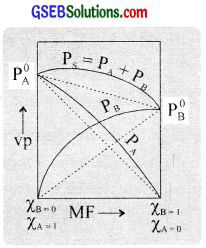Due to the decrease in attractive forces, energy is increased. i.e., the process is endothermic. Thus ∆mixing H > 0 (+ ve). Because of the decrease in attraction, the particles are loosely held and hence there is an increase in volume. Thus ∆mixing V > 0 (+ve). e.g. A solution of ethanol and cyclohexane. In pure ethanol molecules, there arc intermolecular hydrogen bonds.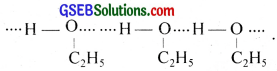When cyclohexane is added to ethanol, the cyclohexane molecules get placed in between the ethanol molecules, thereby breaking the hydrogen bonds. This reduces the intermolecular attraction. As a result, ∆mixing V and ∆mixing H are positive. Solutions such as

• acetone and CS2
• acetone and alcohol
• acetone and benzene
• water and alcohol
• ether and acetone etc. show positive deviation

2. Non – ideal solutions show negative deviations:
In this case the partial vapour pressure of any component of the solution and the total vapour pressure of the solution are less than that expected from Raoult’s law. i.e; PA < XAP0A, PB < XBP0B and Ps < XAP0B. In these solutions ∆mixingH and ∆mixing V are negative.

Cause of negative Deviation:
Negative deviation is shown by liquid pairs of which A – B threes of attraction are stronger than A – A and B – B forces olatiractions. Thus, in the solution the solute and solvent particles are held more tightly than those in the pure components. Due to this, the escaping tendency of the molecules of both the components will be less in the solution than in the pure components. Consequently, the partial pressures of both the components are less titan than expected from Raoult’s law and hence the solution exhibits a less vapour pressure than that expected.Duc to increase in attractive forces. energy is decreased, i.e., the process is exothermic. Thus ∆mixing H < 0 (- ve). Because of the increase in attraction, the particles are tightly held and hence there is a decrease in volume. Thus ∆mixing V < O (- ve). e.g. A solution of chloroform and acetone. When these two are mixed. hydrogen bonding occurs between the two molecular species. This increases the intermolecular attraction as illustrated below.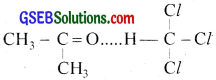This attraction brings their molecules closer thereby reducing the volume. Solutions such as,

• chloroform and ethers
• beniene and chloroform
• waterand nitric acid
• acetone and aniline
• chlorotòrm and nitric acid etc. also show negative deviation.

Azeotropic mixtures:
Mixtures of two liquids which boil ai a constant temperature and can be distilled without any change in their composition arc culled azeotropic mixtures. These are formed by non-ideal solutions and are of two types.

1. Maximum boiling azeotropes:
These are mixtures of two liquids whose boiling points are more than either of the tsso pure components. These are formed by non-ideal solutions showing negative deviations e.g. HNO3 (68 %) + water (32 %) mixture boils at 393.5 K.

2. Minimum boiling azeotropes:
These are mixtures of two liquids whose boiling points are less than either of the two components. These are formed by non-ideal solutions showing positive deviations. e.g. Ethanol (95.6 %) + water (4.4 %) mixture boils at 351.15 K.Question 3.
Vapour pressure – composition graph for a mixture of two substances at a particular temperature is given below.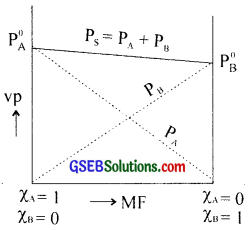(a) Sketch a version of the graph with boiling point as the Y-axis instead of vapour pressure showing the total pressure.
(b) For one of the dotted lines PA ∝ XA (PA & XA are vapour pressure and mole fraction of component A). To turn a proportionality into equality, PA = kXA where k is a constant. Deduce the value of k, when xA = 1
Ideal solutions are those which obey Ruoult’s law over all concentrations and at all temperatures. The properties of ideal solutions are the average of the properties of the components in proportion to their mole fractions. We have seen that for a binary solution of two volatile liquids A and B, the partial pressures of A and B are,
PA = XAP0A and PB = XBP0B

According to Daltons law of partial pressures, the total vapour pressure PS of the solution is given by
PS = PA + PB = XA P0A + XBP0B

According to Raoult’s law. it is obvious that the graph of the partial pressure of each component against its mole fraction should be a straight line. Thus a plot of PA against XA is a straight line passing through P0A When XA = 1 and a plot of PB against X0 is also a straight line passing through P0B When XB = 1. These are represented by dotted lines in the figure. The total vapour pressure of the solution at any composition is equal to the sum of the partial pressures of the two components as shown by the solid hues in the figure.

The essential characteristics of an ideal solution are,

1. Obeys Raoult’s law at all concentrations and temperatures. i.e., PA = XAP0A and PB = XBP0B.
2. The total volume of an ideal solution is equal to the sum of the volumes of the individual components mixed. ∆mixing, V = 0.
3. No. heat is liberated or absorbed in the formation of an ideal solution. i.e., ∆mixing H = 0.

The molecules of the ideal solution will exert sanie force on each other irrespective of their nature. Thus if two liquids A and B from an ideal solution, then the intermolecular forces between A – B, A – A and B – B vill he equal. Sorne examples of ideal solutions are:

• henzene and toluene
• n-hexane and n-heptane
• ethyl bromide and ethyl iodide etc.

Question 4.
The teacher asked Ramu to prepare six solutions from the following compounds

1. C6H6
2. CCl4
3. (CH3)2CO
4. n – hexane
5. C2H5Br
6. C2H5 – I
7. C6H5OH
8. HNO3
9. H2O

He prepared the following solutions.

1. C6H6 + CCl4
2. (CH3)2CO + CCl4
3. C2H5Br + C2H5 – I
4. n – hexane + CH3OH
5. C6H6 + CH3OH
6. HNO3 + H2O

He has to categorise these solutions into three and post them in the respective columns in the given table. How can you help him?Answer: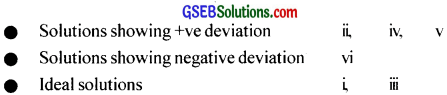Question 5.
The graphical representation of vapour pressure Vs temperature for a liquid and a solution made by dissolving a non-volatile solute in it are given below.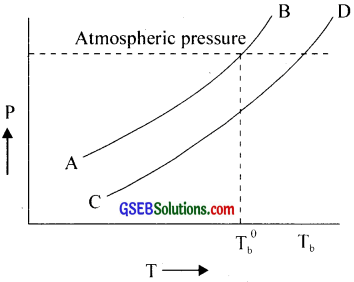Analyse the graph and answer the following questions.

1. Which curve is for solvent?
2. Which curve represents solution?
3. What is meant by ∆Tb?
4. Derive a formula for determining the molecular mass of solute?
5. Write the unit of Kb?

1. AB
2. CD
3. ∆Tb = Tb – T0b

The boiling point of a liquid is the temperature at which the vapour pressure of a liquid becomes equal to atmospheric pressure. When a non-volatile solute is dissolved in a solvent, the vapour pressure is lowered.

Consequently, the temperature at which the vapour pressure becomes equal to the external pressure, will be greater than that of the pure solvent. Therefore, the boiling point of the solution will be higher than that of the solvent. The difference between the boiling points of the solution (Tb) and the solvent (T0b) is called the elevation of boiling point (∆Tb). Thus ∆Tb = Tb – T0b.

For dilute solutions, the elevation of boiling point is directly proportional to the molal concentration of the solute in the solution. Thus, ∆Tb ∝ m or ∆Tb = Kb.m where Kb is a constant called molal elevation constant (ebullioscopic constant) for the solvent.
When m = 1 molal, ∆Tb = Kb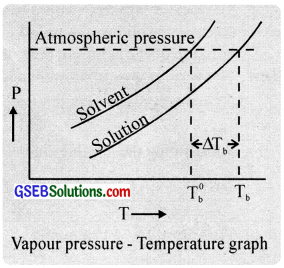The molal elevation constant for the solvent is numerically equal to the elevation in boiling point which is observed for 1 molal solution.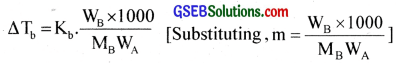As the elevation of boiling point depends only on the number of moles of solute and not on the nature, it is a colligative property.
The molecular mass of solute,This expression is very useful in finding out the molecular mass (Mb) of solutes provided the remaining quantities Kb, ∆Tb WA and WB are known.

Units of Kb
The values of Kb are expressed in degrees/molality or K/m or °C/m or Kkgmol-1

Note: Pressure cooker reduces cooking time: At higher pressure over the liquid (due to weight of the pressure cooker lid), the liquid boils at higher temperature. Therefore, cooking occurs faster.

4. The boiling point of a liquid is the temperature at which the vapour pressure of a liquid becomes equal to atmospheric pressure. When a non-volatile solute is dissolved in a solvent, the vapour pressure is lowered.

Consequently, the temperature at which the vapour pressure becomes equal to the external pressure, will be greater than that of the pure solvent. Therefore, the boiling point of the solution will be higher than that of the solvent. The difference between the boiling points of the solution (Tb) and the solvent (T0b) is called the elevation of boiling point (∆Tb). Thus ∆Tb = Tb – T0b.

For dilute solutions, the elevation of boiling point is directly proportional to the molal concentration of the solute in the solution. Thus ∆Tb ∝ m
or ∆Tb = Kb.m where Kb is a constant called molal elevation constant (ebullioscopic constant) for the solvent.
When m = 1 molal, ∆Tb = KbThe molal elevation constant for the solvent is numerically equal to the elevation in boiling point which is observed for 1 molal solution.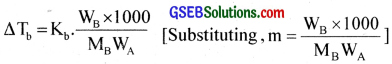As the elevation of a boiling point depends only on the number of moles of solute and not on nature, it is a colligative property.
The molecular mass of solute,This expression is very useful in finding out the molecular mass (Mb) of solutes provided the remaining quantities Kb, ∆Tb WA and WB are known.

Units of Kb: The values of Kb are expressed in degrees/molality or K/m or °C/m or Kkgmol-1

Note: Pressure cooker reduces cooking time: At higher pressure over the liquid (due to the weight of the pressure cooker lid), the liquid boils at a higher temperature. Therefore, cooking occurs faster.

5. Unit of Kb is K kg mol-1Question 6.
The graphical representation of vapour pressure versus temperature for a liquid and a solution made by dissolving a non-volatile solute in it are given below.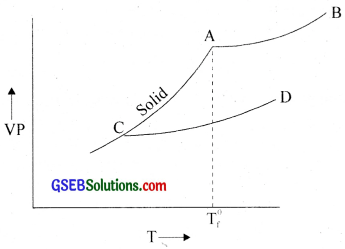Analyse the graph and answer the following.

1. Which curve is for solvent?
2. Which curve is for a solution?
3. How much depression in the freezing point is observed?
4. Derive an expression for calculating the molecular mass of the solute.
5. What is the value of Kf for water?

1. AB
2. CD
3. ∆Tf = T0f – Tf
4. The freezing point of a liquid is defined as the temperature at which the liquid and solid cases of the substance have the same vapour pressure. Since the vapour pressure of a solution is always lower than that of the pure solvent, the vapour pressure of the solution becomes equal to that of the pure solid only at a lower temperature.

As a result, the freezing point of the solution is less than that of the pure solvent. The difference between the freezing
points of the solvent (T0f) and that of the solution (Tf) is known as the depression of freezing point ∆Tf = T0f – Tf of a solution is illustrated graphically as shown in the figure.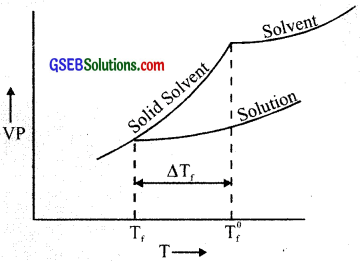For dilute solutions, the depression in freezing point is directly proportional to the molal concentration of the solute in the solution. Thus,
∆Tf ∝ m or ∆Tf = Kfm
where Kf is a constant called molol depression constant (cryoscopic constant) for the solvent.
Substituting. m = 1, ∆Tf = K
Thus, molal freezing point depression constant for the solvent is numerically equal to the depression in freezing point for one molal solution.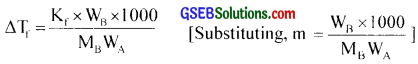As the depression of’ freezing point depends only on the number of moles of solute and solvent and not on the nature of the solute, it is a colligative property. The molecular mass of solute.
MB = $$\frac{K_{f} W_{B} \times 1000}{\Delta T_{f} W_{A}}$$
This expression is of great importance in the calculation of molecular mass of solute (MB) provided the remaining quantities Kf, WB, ∆Tf and WA are known.

Unit of Kf:
The unit of Kf is expressed ¡n degrees/molality or K/m or °C/m or K kgmol-1

Note:

1. Antifreeze solutions:
Water is used in radiators of vehicles. If vehicle is to be used in places where the temperature is less than zero, then the water should freeze in the radiators. The addition of anti-freeze such as ethylene glycol to radiators prevents the freezing of water (due to depression of freezing point). Ethylene glycol is used as a coolant because it is relatively non-volatile (boiling point 470 K) and raises the boiling point of water.

2. Clearing of ice from roads in the hills:
The purpose of spreading NaCl or CaCl2 or various other substances on roadways in the winter season is to depress the freezing point of water and reduce the temperature at which ice is expected to be formed. NaCl can melt ice at a temperature as low as – 21°C. CaCl2 is effective in melting the ice at a temperature as low as – 55°C.

5. Kf for water = 1.86 K mol-1

Question 7.
Does the following picture show the clearing of snow on roads?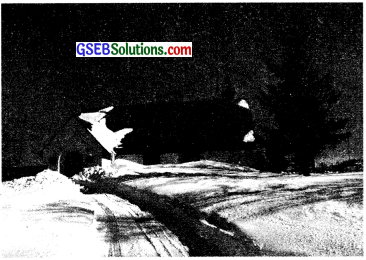(a) Name a chemical useful for removing snow from the road.
(b) Also explain the principle behind it.
1. NaCl
2. Depression in freezing point
The freezing point of a liquid is defined as the temperature at which the liquid and solid cases of the substance have the same vapour pressure. Since the vapour pressure of a solution is always lower than that of the pure solvent, the vapour pressure of the solution becomes equal to that of the pure solid only at a lower temperature.

As a result, the freezing point of the solution is less than that of the pure solvent. The difference between the freezing points of the solvent (T0f) and that of the solution (Tf) is known as the depression of freezing point ∆Tf = T0f – Tf of a solution is illustrated graphically as shown in the figure.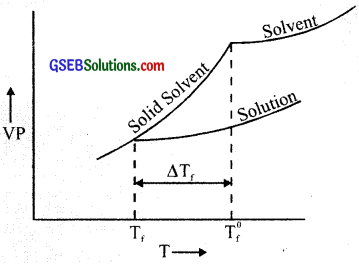For dilute solutions, the depression in freezing point is directly proportional to the molal concentration of the solute in the solution. Thus,
∆Tf ∝ m or ∆Tf = Kfm
where Kf is a constant called molol depression constant (cryoscopic constant) for the solvent.
Substituting. m = 1, ∆Tf = K
Thus, molal freezing point depression constant for the solvent is numerically equal to the depression in freezing point for one molal solution.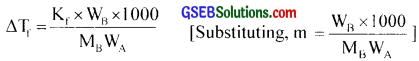As the depression of’ freezing point depends only on the number of moles of solute and solvent and not on the nature of the solute, it is a colligative property. The molecular mass of solute.
MB = $$\frac{K_{f} W_{B} \times 1000}{\Delta T_{f} W_{A}}$$

This expression is of great importance in the calculation of molecular mass of solute (MB) provided the remaining quantities Kf, WB, ∆Tf and WA are known.

Unit of Kf:
The unit of Kf is expressed ¡n degrees/molality or K/m or °C/m or K kgmol-1

Note:

1. Antifreeze solutions:
Water is used in radiators of vehicles. If vehicle is to be used in places where the temperature is less than zero, then the water should freeze in the radiators. The addition of anti freeze such as ethylene glycol to radiators prevents the freezing of water (due to depression of freezing point). Ethylene glycol is used as a coolant, because it is relatively non volatile (boiling point 470 K) and raises the boiling point of water.

2. Clearing of ice from roads in the hills:
The purpose of spreading NaCl or CaCl2 or various other substances on roadways in the winter season is to depress the freezing point of water and reduce the temperature at which ice is expected to be formed. NaCl can melt ice at a temperature as low as – 21°C. CaCl2 is effective in melting the ice at a temperature as low as – 55°C.Question 8.
When dried fruits and vegetables are placed in water they slowly swell. Explain why? What would be the effect of temperature on this process?
Due to osmosis. Movement of water (solvent) from water to the dried vegetables and fruits (solution). On increasing temperature, osmosis increases (Rapid swelling).

Question 9.
It is advised to add ethylene glycol to water in a car radiator while driving on a hill station. Explain?